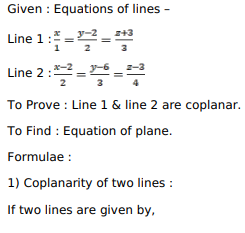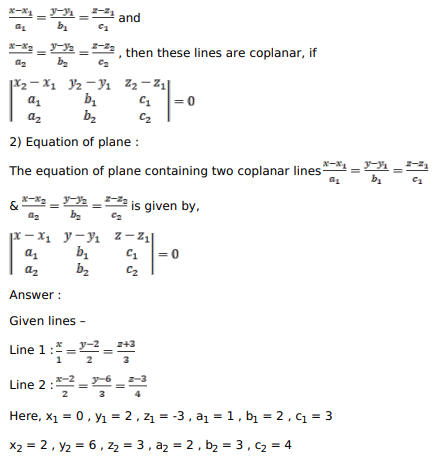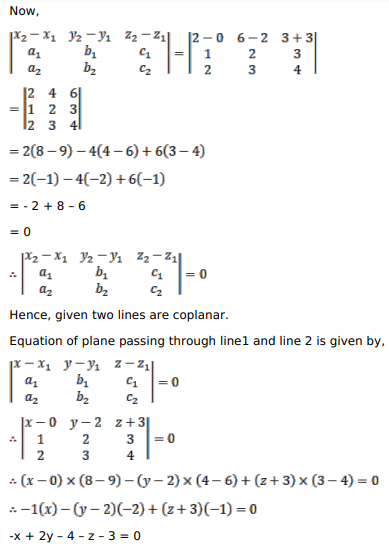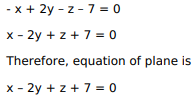# Solve this followingQuestion:

Prove that the lines $\frac{x}{1}=\frac{y-2}{2}=\frac{z+3}{3}$ and $\frac{x-2}{2}=\frac{y-6}{3}=\frac{z-3}{4}$ are coplanar. Also find the equation of the plane containing these lines.

Solution: